Published

# IOTA-Enabled UFO Catcher

Rebuilding the game, so you can waste IOTA coins too.

IntermediateFull instructions provided4 hours1,049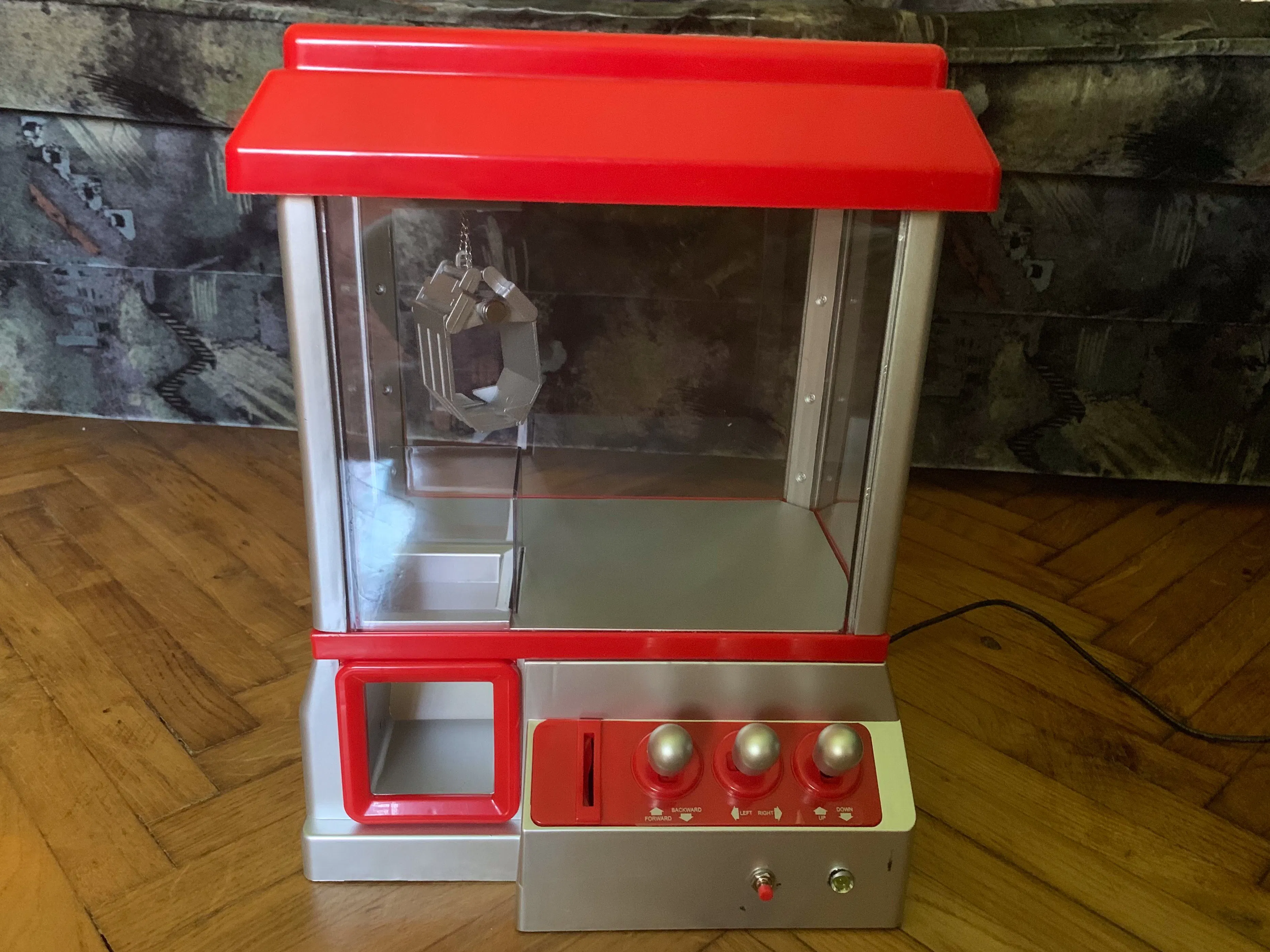## Things used in this project

### Hardware components

 Toy Claw Machine I bought mine at a local store, but it is similar to this one
×1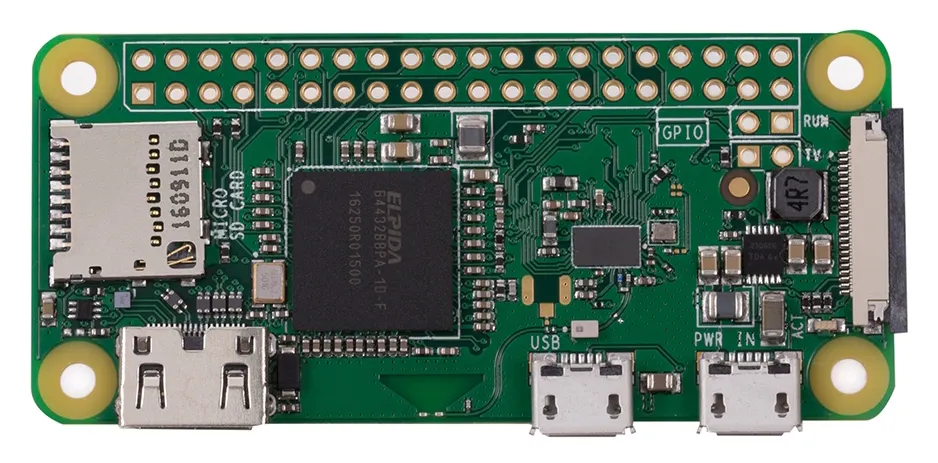Raspberry Pi Zero Wireless I used one for the game and one to serve power.
×2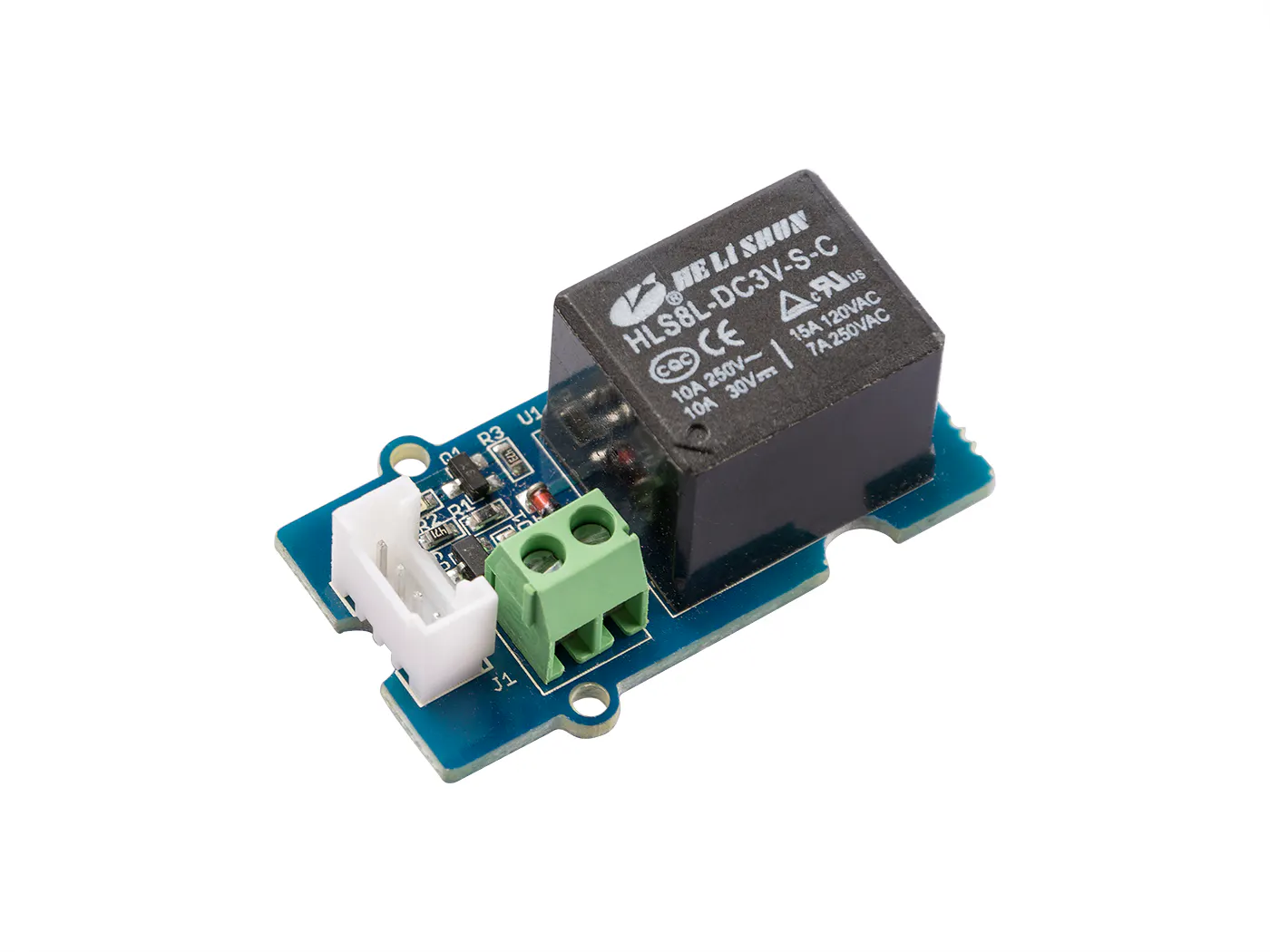Seeed Grove - Relay
×2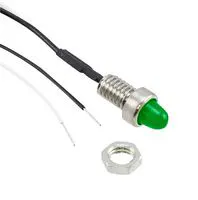LED Panel Mount Indicator, Yellow Green
×1
 Momentary switch
×1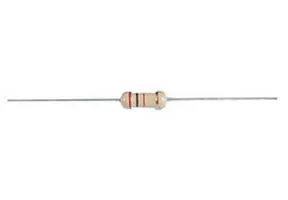Resistor 220 ohm
×2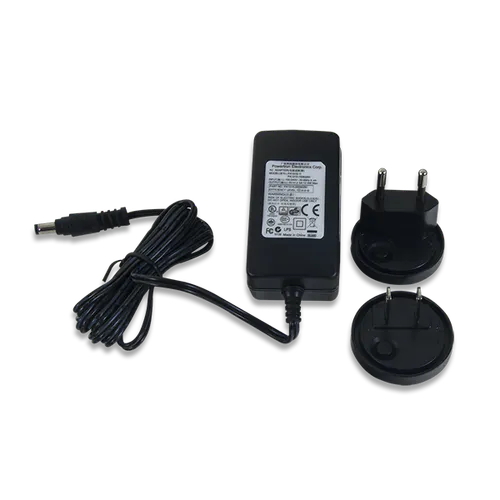Digilent 5V 2.5A Switching Power Supply
×1

### Software apps and online servicesRaspberry Pi RaspbianIOTA Tangle

### Hand tools and fabrication machinesSoldering iron (generic)## Schematics

### Raspberry controlled Power Switch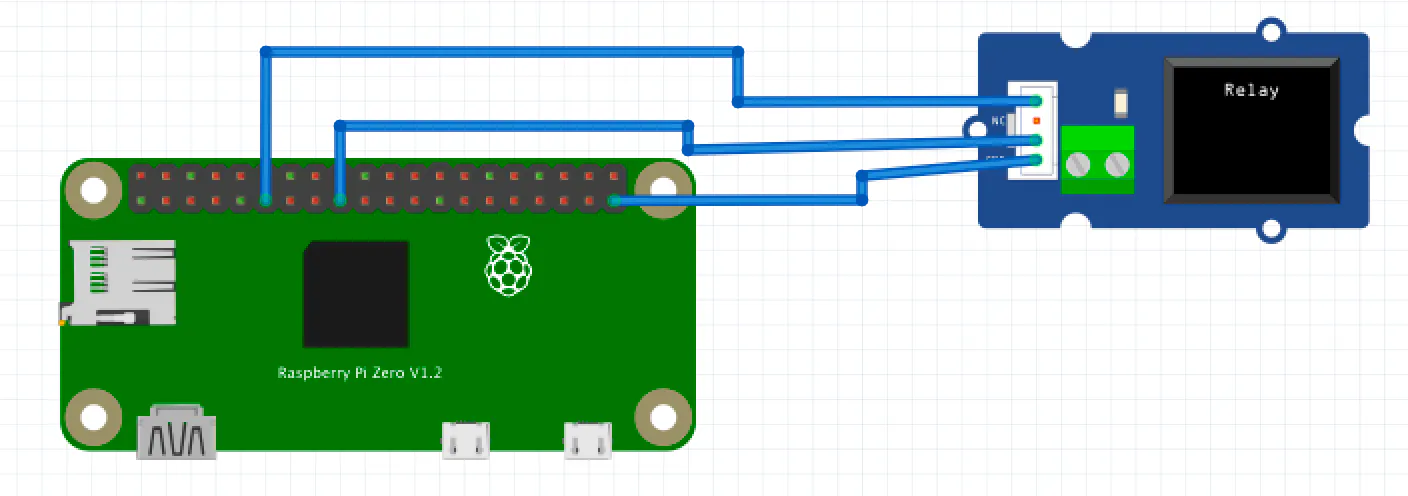### Finished schematics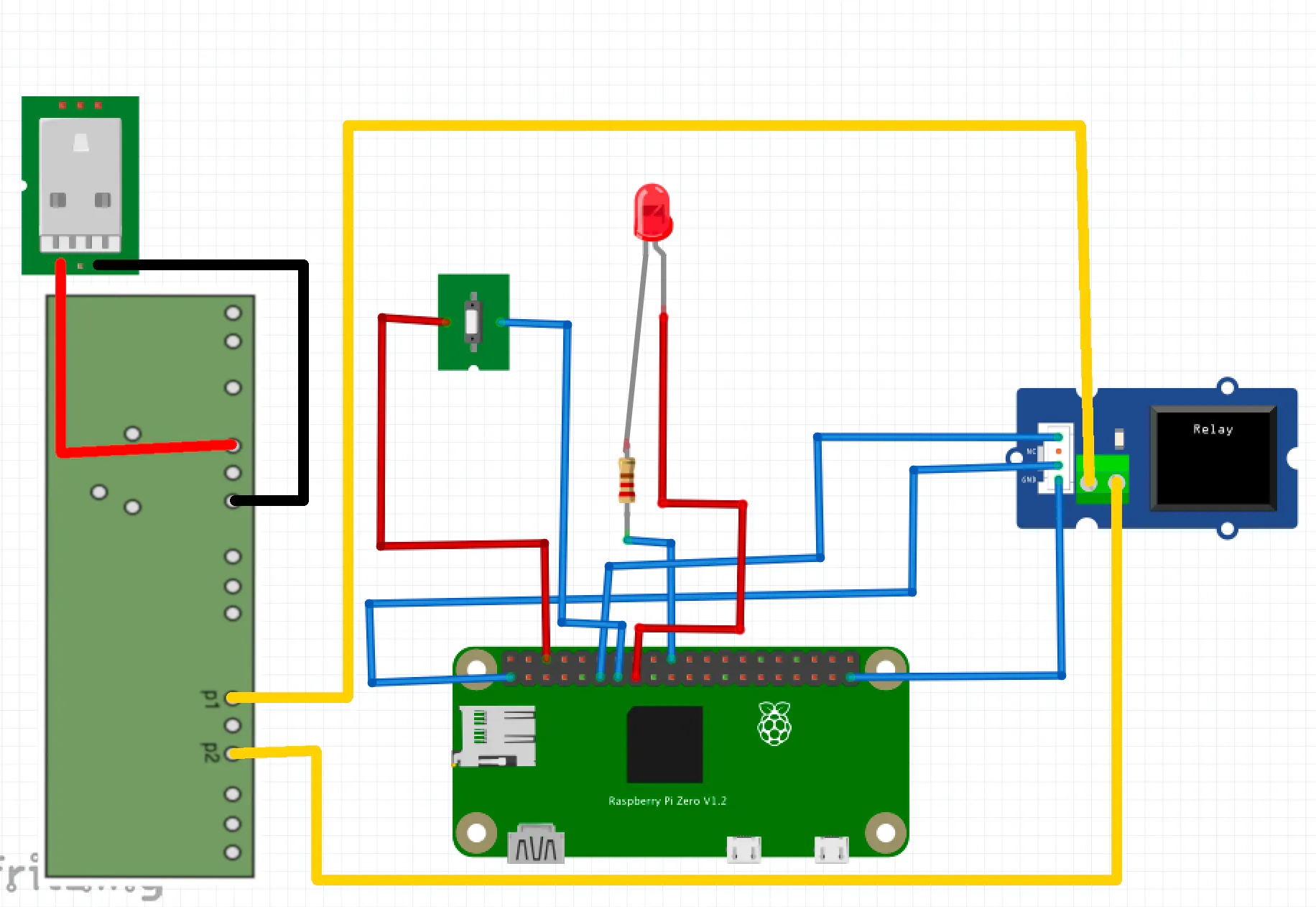## Code

### test.py

Python
Code for testing the completed hardware
```import RPi.GPIO as GPIO
import time

statusPin = 22
buttonPin = 27
relayPin = 17

coins = 1

GPIO.setmode(GPIO.BCM)
GPIO.setwarnings(False)
GPIO.setup(relayPin,GPIO.OUT)
GPIO.setup(statusPin,GPIO.OUT)
GPIO.setup(buttonPin,GPIO.IN,pull_up_down=GPIO.PUD_UP)
GPIO.output(statusPin,GPIO.LOW)

while True:
if coins > 0:
buttonState = GPIO.input(buttonPin)
if buttonState == False:
print "Game-start signal sent"
GPIO.output(relayPin,GPIO.HIGH)
time.sleep(1)
GPIO.output(relayPin,GPIO.LOW)
coins -= 1
if coins > 0:
GPIO.output(statusPin,GPIO.HIGH)
else:
GPIO.output(statusPin,GPIO.LOW)
```

### main.py

Python
Code for the UFO-Catcher
```import RPi.GPIO as GPIO
import time
import sys
import zmq
from iota import Iota
from iota import Transaction
from iota import TryteString
import json

context = zmq.Context()
socket = context.socket(zmq.SUB)

statusPin = 22
buttonPin = 27
relayPin = 17

coins = 0

GPIO.setmode(GPIO.BCM)
GPIO.setwarnings(False)
GPIO.setup(relayPin,GPIO.OUT)
GPIO.setup(statusPin,GPIO.OUT)
GPIO.setup(buttonPin,GPIO.IN,pull_up_down=GPIO.PUD_UP)
GPIO.output(statusPin,GPIO.LOW)

def button_processor():
global coins
while True:
if coins > 0:
buttonState = GPIO.input(buttonPin)
if buttonState == False:
print "Game-start signal sent"
print "New balance: " + str(coins)
GPIO.output(relayPin,GPIO.HIGH)
time.sleep(1)
GPIO.output(relayPin,GPIO.LOW)
coins -= 1
if coins > 0:
GPIO.output(statusPin,GPIO.HIGH)
else:
GPIO.output(statusPin,GPIO.LOW)

def payment_processor():
seed = 'SEED'
p_api = Iota('https://nodes.devnet.iota.org:443', seed)
while True:
tx = ProposedTransaction(
message=TryteString.from_unicode('Power Socket payment.'),
tag=Tag('POWERSWITCHCOIN'),
value=1
)
time.sleep(60)

tx = api.prepare_transfer(transfers=[tx])

result = api.send_trytes(tx['trytes'], depth=3, min_weight_magnitude=9)

socket.setsockopt(zmq.SUBSCRIBE, b'tx')
socket.connect('tcp://zmq.devnet.iota.org:5556')

x.start()
y.start()

while True:
try:
topic, data = socket.recv().decode().split(' ', 1)
if topic == 'tx':
tx_hash, address, value, obs_tag, ts, index, lastindex, bundle_hash, trunk_hash, branch_hash, received_ts, tag = data.split(' ')
coins += int(value)
print "New balance: " + str(coins)
except KeyboardInterrupt:
print("Stopping now")
GPIO.output(statusPin,GPIO.LOW)
GPIO.output(relayPin,GPIO.LOW)
x.join()
y.join()
sys.exit()
```

### requirements.txt

BatchFile
Python requirements
```PyOTA==2.0.7
pyzmq==18.0.1
```

### powerswitch.py

Python
Code for power supply
```import RPi.GPIO as GPIO
import time
import sys
from iota import Iota
import pprint
import json

relayPin = 17

timeRemaining = 0
lastBalance = 0
seed = 'SEED'
api = Iota('https://nodes.devnet.iota.org:443', seed)

GPIO.setmode(GPIO.BCM)
GPIO.setwarnings(False)
GPIO.setup(relayPin,GPIO.OUT)

while True:

if timeRemaining > 0:
timeRemaining -= 1
GPIO.output(relayPin,GPIO.HIGH)
else:
GPIO.output(relayPin,GPIO.LOW)
x = api.get_account_data()
balance = int(x["balance"])
change = balance - lastBalance
timeRemaining += change
time.sleep(60)
```